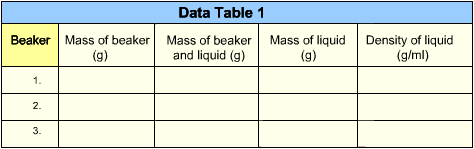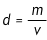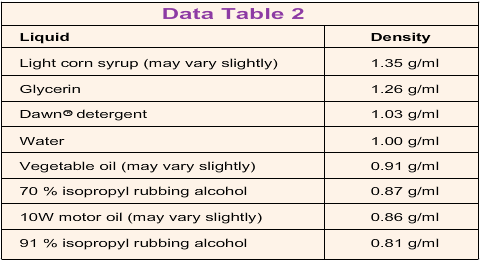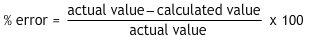# CHEMISTRY

## Density of Liquids

1
2
3
Unknown 1
Unknown 2
Unknown 3Introduction
Materials
Instructions

Objects that are the same size often have different masses. For example, a small cube of metal is much heavier than a cube of wood the same size. That is because metal is more dense than wood. Even though two objects may have the same dimensions, their density can vary greatly. Density (d) is calculated by dividing an object’s mass (m) by its volume (v):Liquids, even two liquids that look similar, can be distinguished by their densities. The density of a liquid can be found by determining the mass of a sample of the liquid and dividing it by the volume. In this experiment, you will identify three unknown liquids by their densities.

Density is a physical characteristic of a material, just like color or shape. Because the density of a substance is constant, it is often used in chemistry to determine the composition or identity of an unknown material. Since density is calculated by dividing the mass by the volume of a sample of matter, its actual value is not dependent on sample size. A 10-ml sample should have the same density as a 100-ml sample because the difference in mass will be proportional to the difference in volume.

When attempting to identify substances by their densities, there are many possible sources of error. A scale or triple-beam balance that is not properly calibrated or a dirty beaker can cause serious discrepancies in the calculation. In addition, impurities found in a sample can change the mass of the sample, so an incorrect density could be calculated. The calculated density may also be affected if the volume is not measured as precisely as possible. A volumetric flask or graduated cylinder may be used to make the measurement more accurate.

• Three 100-milliliter(ml) beakers
• Scale
• Unknown liquid 1
• Unknown liquid 2
• Unknown liquid 3
1. Find the mass of each beaker in grams (g). Record the mass on Data Table 1. After filling each cell, click the diskette icon in the bottom menu bar to continue.
2. Pour 50 ml of an unknown liquid into beaker 1. Repeat with two other unknown liquids in beakers 2 and 3.
3. Find the total mass of beaker 1 and the liquid in it. Record this measurement on Data Table 1. After filling each cell, click the diskette icon in the bottom menu bar to continue.
4. Find the mass of the liquid in beaker 1 by subtracting the mass of the beaker from the total mass. Record your calculation on Data Table 1. After filling each cell, click the diskette icon in the bottom menu bar to continue.
5. Repeat steps 3 and 4 with beakers 2 and 3.
6. Calculate the density of each liquid by dividing its mass by its volume (50 ml). Record the density of each liquid on Data Table 1. After filling each cell, click the diskette icon in the bottom menu bar to continue.
7. Click the table icon in the bottom menu bar to access Data Table 2. It shows the densities of some common liquids. Use this table to determine the identity of liquids 1, 2, and 3.
TableCalculator
C
7 8 9 + 4 5 6 - 1 2 3 ÷ 0 . = x
Analysis
3.